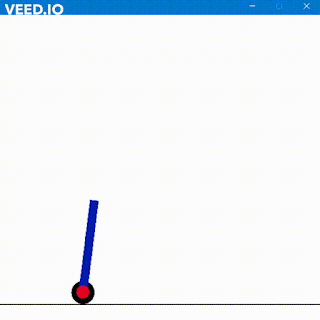## JK Flip Flop Truth Table

has many advantages, but it also comes with various disadvantages. Among them is the forbidden condition whose result can either be 1 or 0. Thus to avoid this condition JK Flip Flop comes in rescue.

JK Flop Flop is also a universal flip-flop and has the same input as compared to SR Flip Flop. The inputs also are fed with a clock signal which in JK Flip Flop is level triggered and not edge triggered. Both S and R are replaced by J and K in the below basic diagram of JK Flip Flop. The input nand gates are 3-input based rather than 2 inputs based on the SR Flip Flop.

Since JK is supplied with the feedback thus it eliminates the forbidden condition.

Here is the truth table

 CLK J K Q Q’ Condition 1 0 0 0 1 No Change 0 0 0 0 1 No Change 1 0 1 1 1 Reset 0 0 1 0 0 Reset 1 1 0 0 1 Set 0 1 0 1 0 Set 1 1 1 0 1 Toggle 0 1 1 1 0 Toggle

The toggle action takes place whenever both inputs are 1.
Now when J = 0 and K = 0 and CLK = 1 Q is 0 and for CLK = 0 Q is still 0 thus No Change.

When J = 0 and K = 1 and CLK = 1 Q is 1 and for CLK = 0 Q is 0 thus Reset to 0.

When J = 1 and K = 0 and CLK = 1 Q is 0 and for CLK = 0 Q is 1 thus Set to 1.
When J = 1 and K = 1 and CLK = 1 Q is 0 and for CLK = 0 Q is 1 thus Toggle.

JK FF avoids the forbidden condition, however, even JK cannot escape the Race Around Condition. The Master-Slave is basically two JK Flip Flops in series together. The clock is fed into the master flip-flop and the inverted clock is fed into the slave flip-flop. When master acts slave is locked so while the master is changing its value output doesn’t change and during the other level of clock, slave is changing because of changed master output. Now the master is locked thus to prevent input change. After this cycle output and input are allowed to change.

What is Race around Condition?

Race around the condition in JK flip flop occurs when J = 1 and K = 1 but the output keeps toggling between 0 and 1 instead of changing only once while clock is 1. The time interval of oscillation is the delay of the circuit. Thus to prevent this toggling Master Slave bistable JK Flip Flop is used.

Some of the ICs regarding JK flip flops are 74LS73, 74LS107 etc dual JK flip flop

Verilog Code for JK Flip Flop with Race Around Condition.

Output Waveform

Now if we chose Q = 0 then J=0 K=0 Q(n+1) = Q (here Q = 0)
For J=0 K=0 Q(n+1)=0
For J=0 K=0 Q(n+1)=1
For J=1 K=1 Q(n+1)=Q' (here Q = 0 thus Q' will be 1)

Now Q' should be equal to but one can see in the output that for J=1 and K=1 the output keeps toggling between  0 and 1 while clock is 0. Similarly in the duration for which clock remains 1 Q must retain a single value however is toggling continuously (in the red box). THIS IS CALLED RACE AROUND CONDITION.

Thus avoid this condition we use Master Slave JK Flip Flop.

In Master Slave JK we provide clock master and the complement of the clock to slave. So the procedure is when posedge of the clock is applied the master is driven and salve is locked since it gets the complement of clock i.e. negedge. Hence Output remains stable. When negedge of the clock is applied the Master is locked and inputs are stable while the slave declares its output. Thus one might find a delay between J-K driving point and Q and Qbar output point.

### The running Output

Observe when J=0 and K=0/1 Q becomes 0 and Q' becomes 1 and for J=1 and K=0 Q becomes 1 and For J = K = 1 Q toggles

Feel free to contact for any suggestions

We would love to hear from you.

#### 1 comment:

1.Pls upload this code...jkff with race around condition

### Self Balancing Robot using Machine Learning

Hola Amigos, I have always loved inverted pendulums. They are very fascinating to me and I play with them a lot. In real life, I have m...